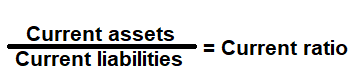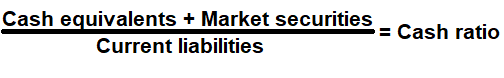# Liquidity Ratio Analysis

Liquidity ratio is a financial metrics that is used to determine a company's ability to pay short and long-term liabilities (Loans/Bills payable) without raising external capital. Business Owners, Managers, Investors and Financial lenders use it to measure a company's ability to pay short and long-term liabilities with its cash or quick assets.

A company may fall in liquidity crunch if the bills payable are due before its receives its money. Liquidity ratios are most useful when they are used in comparative form. The following metrics is used commonly to measure and manage a business:

# Current Ratio

Financial metric used to measure a company's ability to pay off its short-term liabilities with its current assets. In other words, the ratio calculates number of times a company pay off its current liabilities, using its current assets.

A company can easily convert its current assets into cash in short term to pay off its current liabilities if required.

### Formula:### How to use this equation?

This is a balance sheet component; the values are commonly stated against current assets and current liabilities. To use this ratio, simply divide current assets with current liabilities.

Current Assets:
On the balance sheet, you will find the total value of current assets which consist of cash, cash equivalents, account receivables, merchandise inventory and marketable securities.

Current Liabilities:
On the balance sheet, you will find the total value of current liabilities which consist of accounts payable, tax payable (sales, payroll and other taxes) that are due in short-term.

Example:
Current ratio for a company with total current assets of \$200,000 and current liabilities of \$155,000 is 1.29:1. It means \$1.29 of current assets are available to cover each \$1 of its current liabilities.

# Quick Ratio

Financial metric is used to measure, if the company has enough quick assets (current assets less inventory and prepaid expenses) readily available to pay off its current liabilities. In other words, the ratio calculates number of times a company could pay off the debt that's due within the next 12 months using current assets other than inventory.

It will be smaller than the current ratio, but it's still important to consider because it shows whether a company has enough cash and other assets to quickly turn into cash to pay off debts owed.

### Formula:### How to use this equation?

This is a balance sheet component; the values are commonly stated against current liabilities, cash, cash equivalents, account receivables, merchandise inventory and marketable securities. To use this ratio, subtract inventory and prepaid expenses from current assets and then divide it with current liabilities.

Current Assets:
On the balance sheet, you will find the total value of current assets which consist of cash, cash equivalents, account receivables, merchandise inventory and marketable securities.

Inventory:
Inventory is the finished goods that can be sold immediately.

Current Liabilities:
On the balance sheet, you will find the total value of current liabilities which consist of accounts payable, tax payable (sales, payroll and other taxes) that are due in short-term.

Example:
Quick ratio for a company with total current assets of \$240,000, merchandise inventory of \$40,000 and current liabilities of \$105,000 is 1.9:1. It means \$1.9 of quick assets available to cover each \$1 of short-term debt (current liabilities).

# Cash Ratio

Financial metric utilizes only the most liquid of assets - cash equivalents and marketable securities to pay off its current liabilities. In other words, in order to pay off its current debt, the company would have to use all of its cash equivalents and marketable securities.

A higher cash coverage ratio means that the company is more liquid and can more easily fund its debt.

### Formula:### How to use this equation?

This is a balance sheet component; the values are commonly stated against current liabilities and cash equivalents, marketable securities under current assets. To use this ratio, add cash equivalents and marketable securities from current assets and then divide it with current liabilities.

Cash Equivalents:
Cash equivalents are any short-term investment securities that have maturity periods of 90 days or less. These include bank certificates of deposit, banker's acceptances, treasury bills, commercial paper, and other money market instruments.

Marketable securities:
Investments in common stock, preferred stock, corporate bonds, or government bonds, short-term commercial paper and certificates of deposit (CDs) that can be readily sold or redeemed.

Current Liabilities:
On the balance sheet, you will find the total value of current liabilities which consist of accounts payable, tax payable (sales, payroll and other taxes) that are due in short-term.

Example:
Cash ratio for a company with a cash equivalents of \$10,000, marketable securities of \$20,000 and current liabilities of \$50,000 is 0.6:1. It means \$0.60 of cash equivalents and marketable securities available to cover each \$1 of short-term debt (current liabilities).

# Net Working Capital to Total Assets

Financial metric is used to analyze the extent of assets tied up in working capital, or the amount of assets required to run the day to day operations of a company. In other words, it is used to measure the net liquid assets of a company as a percentage of its total assets.

An increasing ratio is considered good, since it implies that a business is minimizing its investment in fixed assets and keeping its asset reserves as liquid as possible.

However, ratio can be negative, indicating that current assets are greater than current liabilities. In general, a negative ratio is viewed as a sign that the business is in financial distress and does not have the necessary liquid assets to pay its current liabilities as they fall due.

### Formula:### How to use this equation?

This is a balance Sheet component; the values are commonly stated against Current Assets, Current Liabilities and Total Assets. To use this ratio, First obtain the working capital value by subtracting current liabilities from current assets and then divide it with total assets.
Note:
Net Working Capital = total current assets - total current liabilities.
It is a liquidity calculation that measures a companyâ€™s ability to pay off its current liabilities with current assets.

Current Assets:
This is a balance sheet component - cash, cash equivalents, account receivables, merchandise inventory and marketable securities can easily be converted into cash in the short term.

Current Liabilities:
This is a balance sheet component - accounts payable, tax payable (sales, payroll and other taxes) that are due in short-term.

Total Assets:
Total assets are the sum of all current and noncurrent assets that a company owns.

Example:
Net working capital ratio for a company with total current assets of \$180,000, current liabilities of \$85,000 and total assets of \$220,000 is 0.43:1 or 43% indicating that the company has additional liquidity and financial strength to meet its working capital requirement.